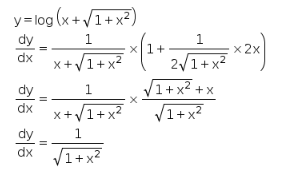# If y = log (x + (1 + x2)1/2) prove that dy/dx = 1/(1 + x2)1/2

if y = log (x + (1 + x2)1/2)
prove that dy/dx = 1/(1 + x2)1/2